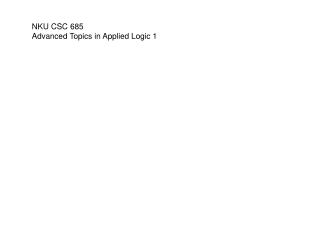DownloadDownload PresentationNKU CSC 685 Advanced Topics in Applied Logic 1

# NKU CSC 685 Advanced Topics in Applied Logic 1

Télécharger la présentation## NKU CSC 685 Advanced Topics in Applied Logic 1

- - - - - - - - - - - - - - - - - - - - - - - - - - - E N D - - - - - - - - - - - - - - - - - - - - - - - - - - -
##### Presentation Transcript

1. NKU CSC 685 Advanced Topics in Applied Logic 1

2. while (...) { ... csec { ... } .... } thread 1 thread 2 More LTL examples: Finite state processes G(start  ready) G(request  F ack) GF enabled FG deadlocked G((floor2  up  pressed5)  (up U floor5)) Critical section example "" over paths not directly expressible States={non-critical, trying, critical} "" over paths not expressible Safe: M,q |= G(c1c2) Live: M,q |= G(t1Fc1) Non-Blocking: every n state has a path that later has a t state No Strict Sequencing: notM,q |= G(c1  c1W (c1  c1Wc2 )

3. req=T status=ready req=T status=busy H&R Fig 3.9,10 req=F status=busy req=F status=ready MODULE main VAR req : boolean; status : {ready,busy}; ASSIGN init(status) := ready; next(status) := case req : busy; 1 : {ready,busy}; esac; LTLSPEC G(req -> F status=busy) NuSMV code with a Linear Temporal Logic Specification

4. MODULE main VAR pr1: process prc(pr2.st, turn, 0); pr2: process prc(pr1.st, turn, 1); turn: boolean; ASSIGN init(turn) := 0; -- safety LTLSPEC G!((pr1.st = c) & (pr2.st = c)) -- liveness LTLSPEC G((pr1.st = t) -> F (pr1.st = c)) LTLSPEC G((pr2.st = t) -> F (pr2.st = c)) -- `negation' of strict sequencing (desired to be false) LTLSPEC G(pr1.st=c -> ( G pr1.st=c | (pr1.st=c U (!pr1.st=c & G !pr1.st=c | ((!pr1.st=c) U pr2.st=c)))))

5. MODULE prc(other-st, turn, myturn) VAR st: {n, t, c}; // = {non-critical, trying, critical} ASSIGN init(st) := n; next(st) := case (st = n) : {t,n}; (st = t) & (other-st = n) : c; (st = t) & (other-st = t) & (turn = myturn): c; (st = c) : {c,n}; 1 : st; esac; next(turn) := case turn = myturn & st = c : !turn; 1 : turn; esac; FAIRNESS running FAIRNESS !(st = c)

6. partial correctness if before P starts  is true and P halts then after P halts  is true code in a procedural language with: ; (sequencing) if/else while variables of type integer,+,-,*,/,=,< pre post par first order logic formulas with +,-,*,/,=,< no variables also appearing in P are bound proof system Partial correctness logic |= par (|  |)P(|  |) Hoare triple

7. pre post i=n  n > 0 s = n(n+1)/2 Partial correctness logic: Example s=0; while ( i > 0 ) { s= s + i ; i= i - 1 ; } |= par (|  |)P(|  |)

8. Proof Rules for Partial Correctness ;-introduction (||) C1 (||), (||) C2(||) |par (||) C1;C2(||) =-introduction |par (|[E/x]|) x=E (||) if-introduction (|B|) C1 (||), (|B|) C2(||) |par (||) if B {C1} else {C2} (||) while-introduction (|B|) C1 (||) |par (||) while B {C} (|B|) arithmetic implication arith, (||) C (||),  arith |par(||) C (||)